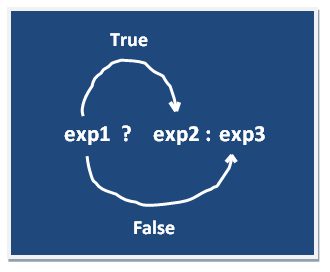# Find Greatest Number Program in C++

## Using if Statements

```
#include<conio.h>
#include<iostream.h>

void main()
{
clrscr();
int a, b, c;
cout <<"Enter any three numbers: ";
cin>>a;
cin>>b
cin>>c;
if(a>=b && a>=c)
{
cout<<"Largest number: "<<a;
}
if(b>=a && b>=c)
{
cout<<"Largest number: "<<b;
}
if(c>=a && c>=b)
{
cout<<"Largest number: "<<c;
}
getch();
}
```

## Output

```Enter any three numbers:
15
30
20
Largest number: 30
```

## Ternary operator

If any operator is used on three operands or variable is known as ternary operator. It can be represented with " ?: "In the above symbol exp1 is condition and exp2 and exp3 will be either value or variable or statement or any mathematical expression. If condition will be true exp2 will be executer otherwise exp3 will be executed.

## Using Ternary Operator

```
#include<iostream.h>
#include<conio.h>

void main()
{
int a,b,c,largest;
clrscr();
cout<<"Enter any three numbers: ";
cin>>a>>b>>c;
largest=(a>b)?(a>c?a:c):(b>c?b:c);
cout<<"Largest number: "<<largest;
getch();
}
```

## Output

```Enter any three numbers:
5
10
4
Largest number: 10
```

## Pure VPN Privide Lowest Price VPN Just @ \$1.65. Per Month with Non Detected IP Lowest Price Non Detected IP VPN